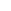# DedeCMS织梦模板下载添加一个实现任意字段调用的函数

10-02 46阅读

function table(\$table, \$field, \$id) {         global \$dsql;         \$primarys = array();         \$table = strpos(\$table, '#@_') === false?(strpos(\$table, 'dede_') ===false?''.\$table:str_replace('dede_','',\$table)):\$table;         \$dsql -> Execute("me","SHOW COLUMNS FROM `\$table`");         while (\$r = \$dsql->GetArray())         {                 if(\$r['Key'] == 'PRI') \$primarys[] = \$r['Field'];         }         if(!empty(\$primarys))         {                 \$primary = \$primarys;                 \$result = \$dsql -> GetOne("SELECT `\$field` FROM `\$table` WHERE `\$primary`= \$id");         }         return isset(\$result[\$field])?\$result[\$field]:''; }

{dede:标记 function='table("要调用的表名","要调用的字段",@me)'/}

[field:字段 function=table("要调用的表名","要调用的字段",@me)/]

{dede:arclist row='10' titlelen='24'} [field:id function=table('addonarticle','body',@me)/] {/dede:arclist}

## 还没有评论，来说两句吧...